Equivalent Fractions

Chapter 7 Class 6 Fractions
Concept wise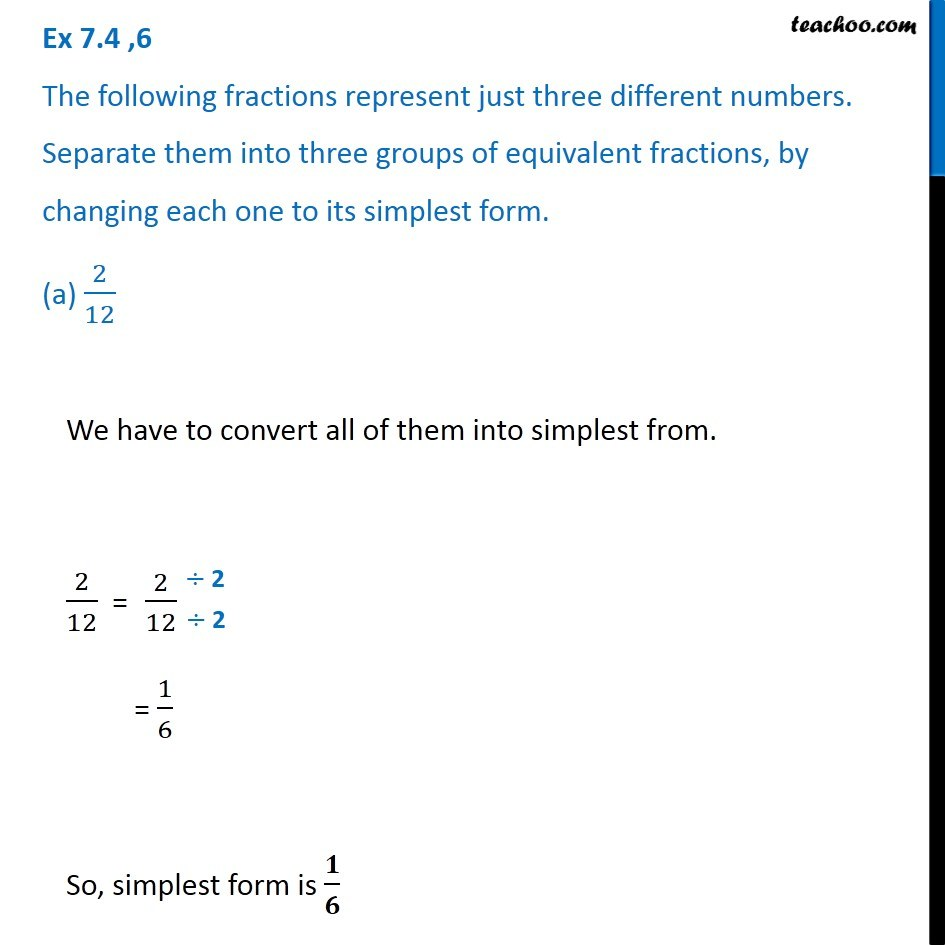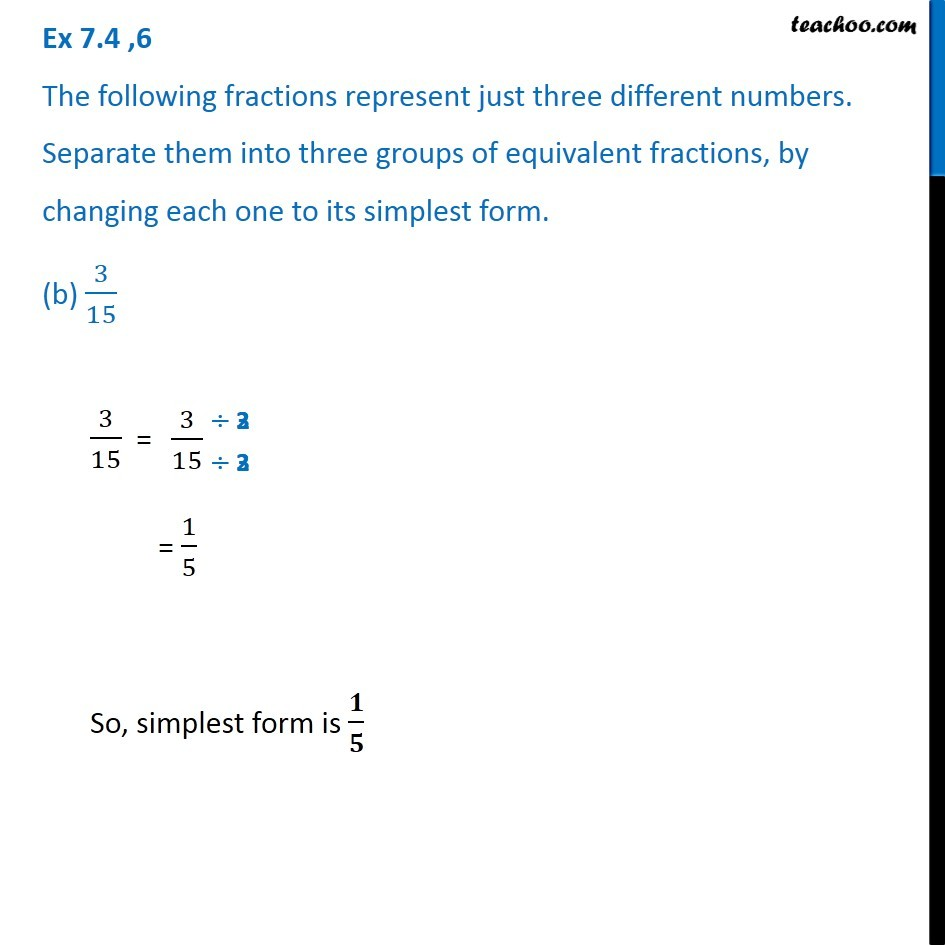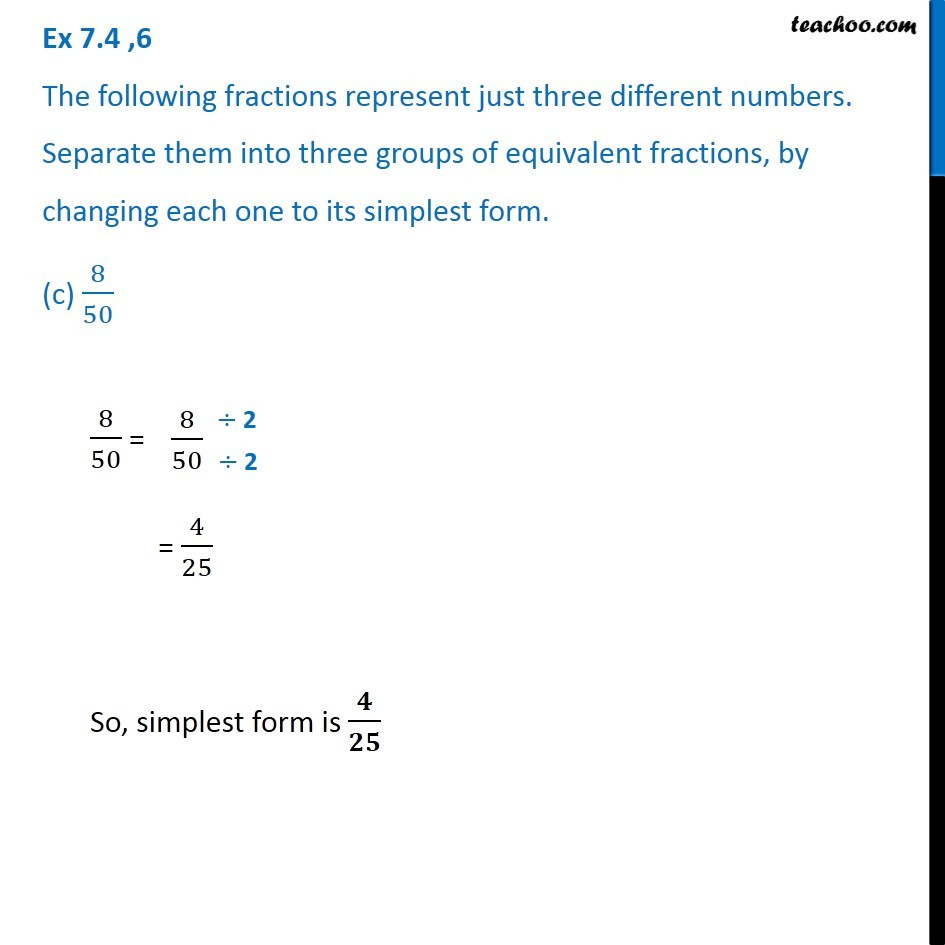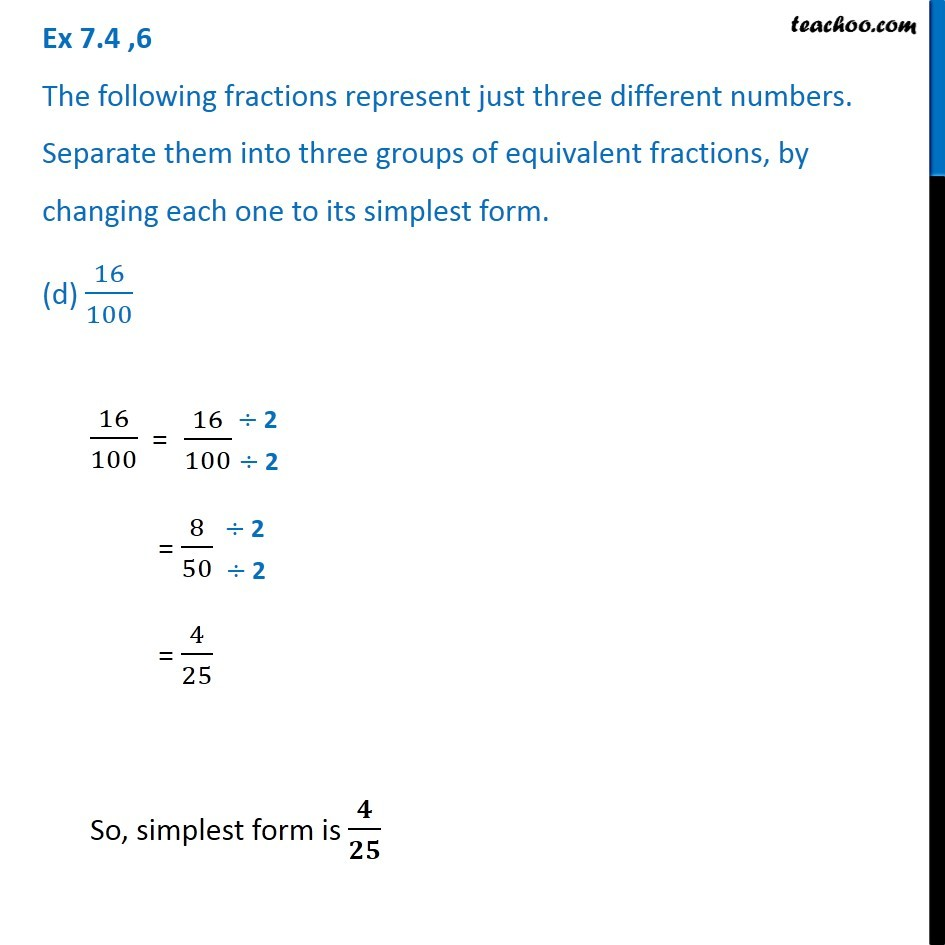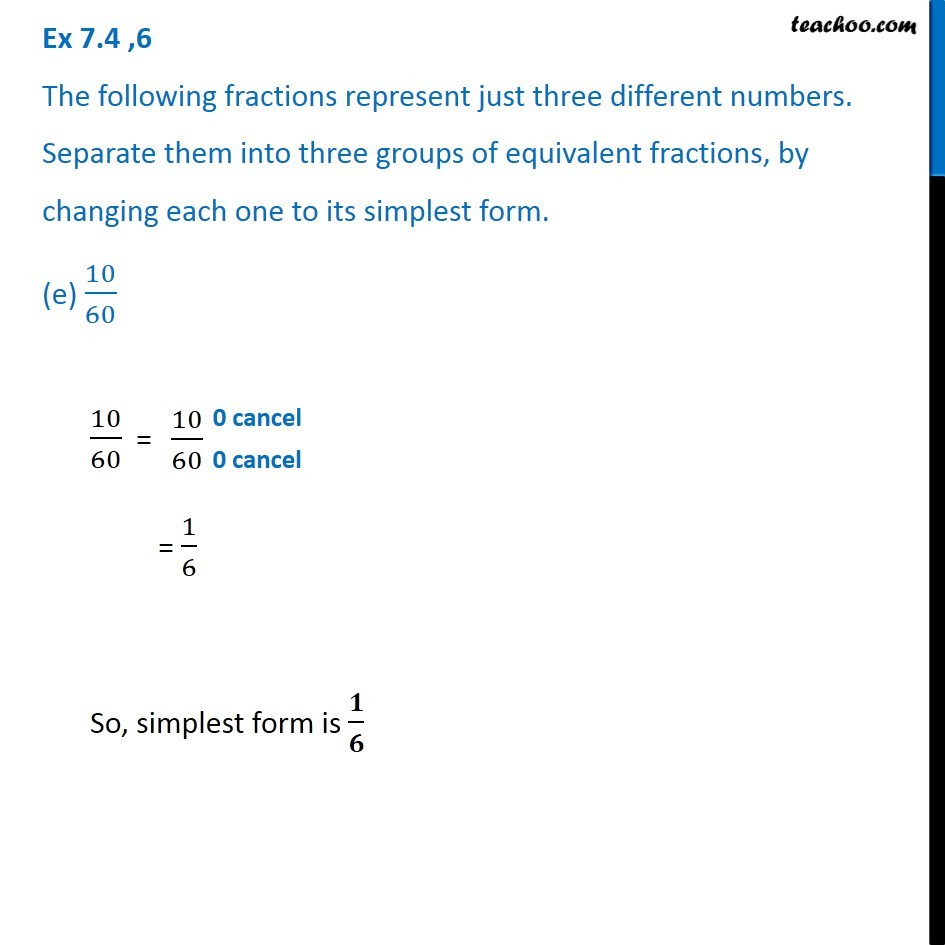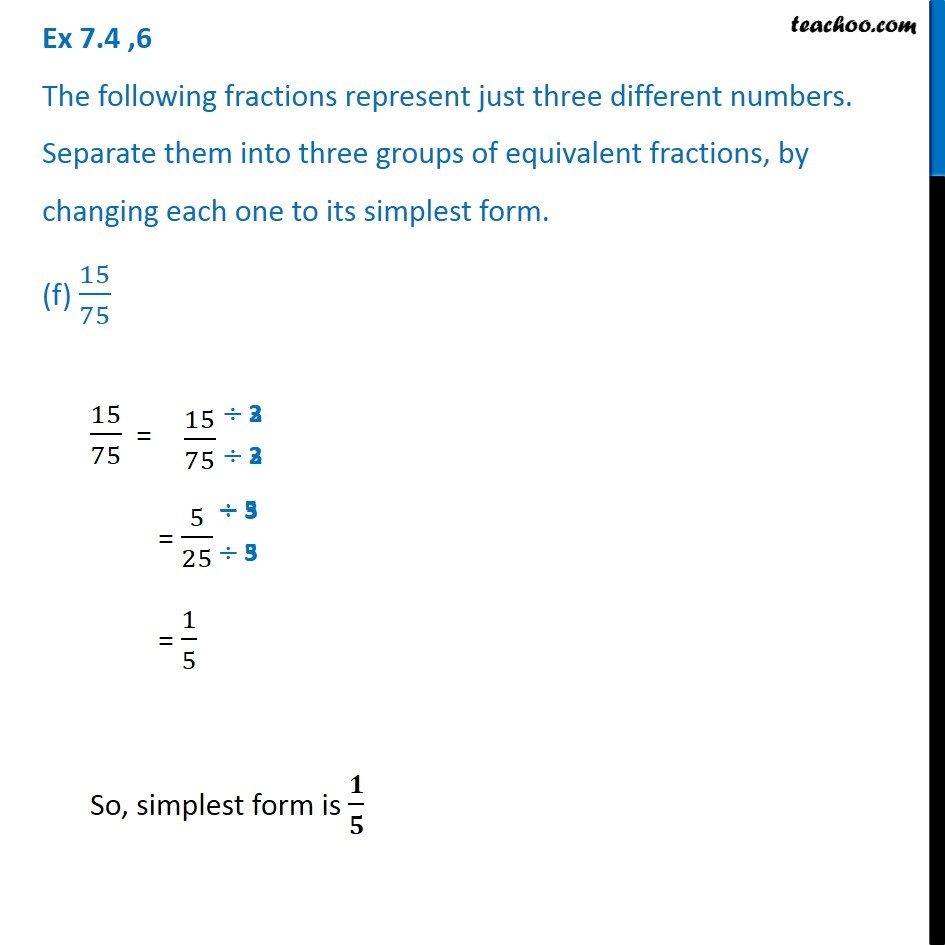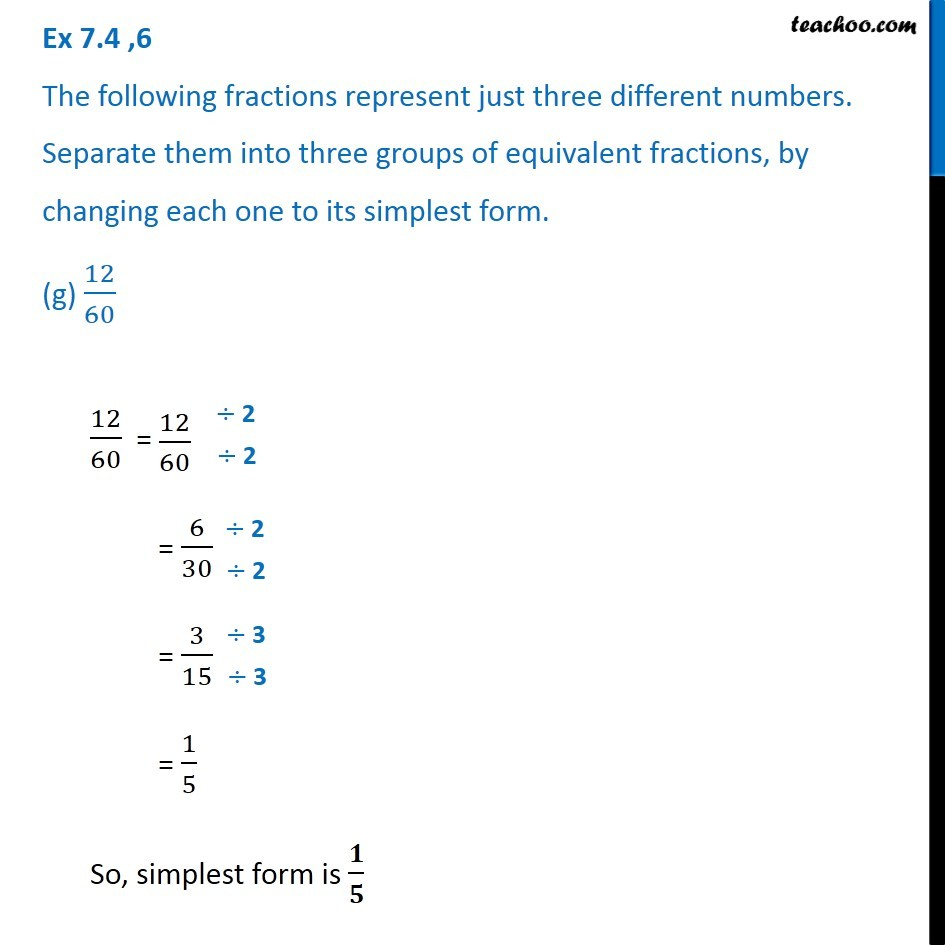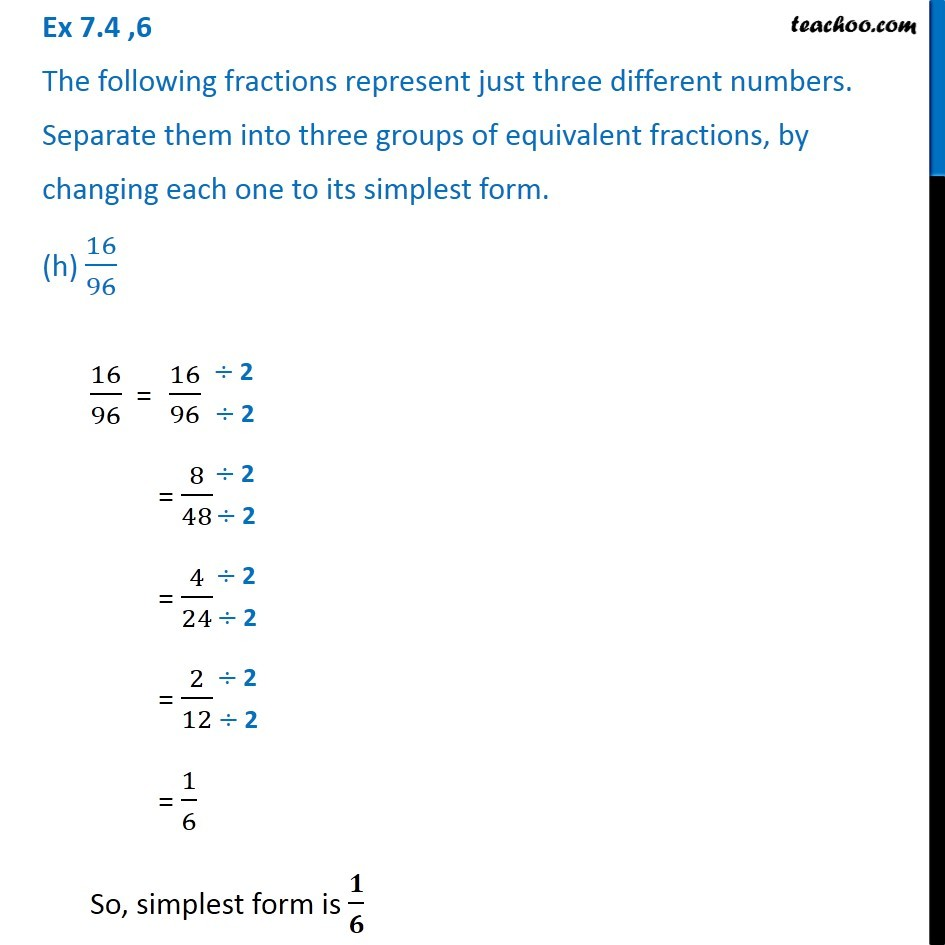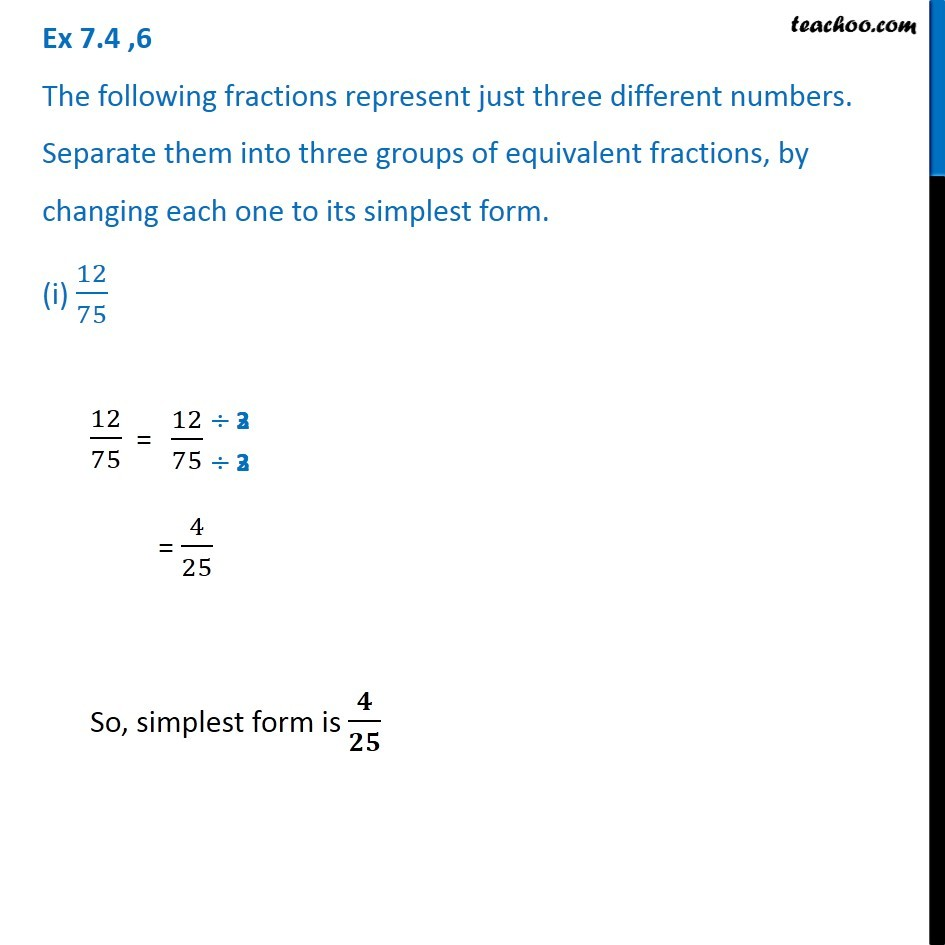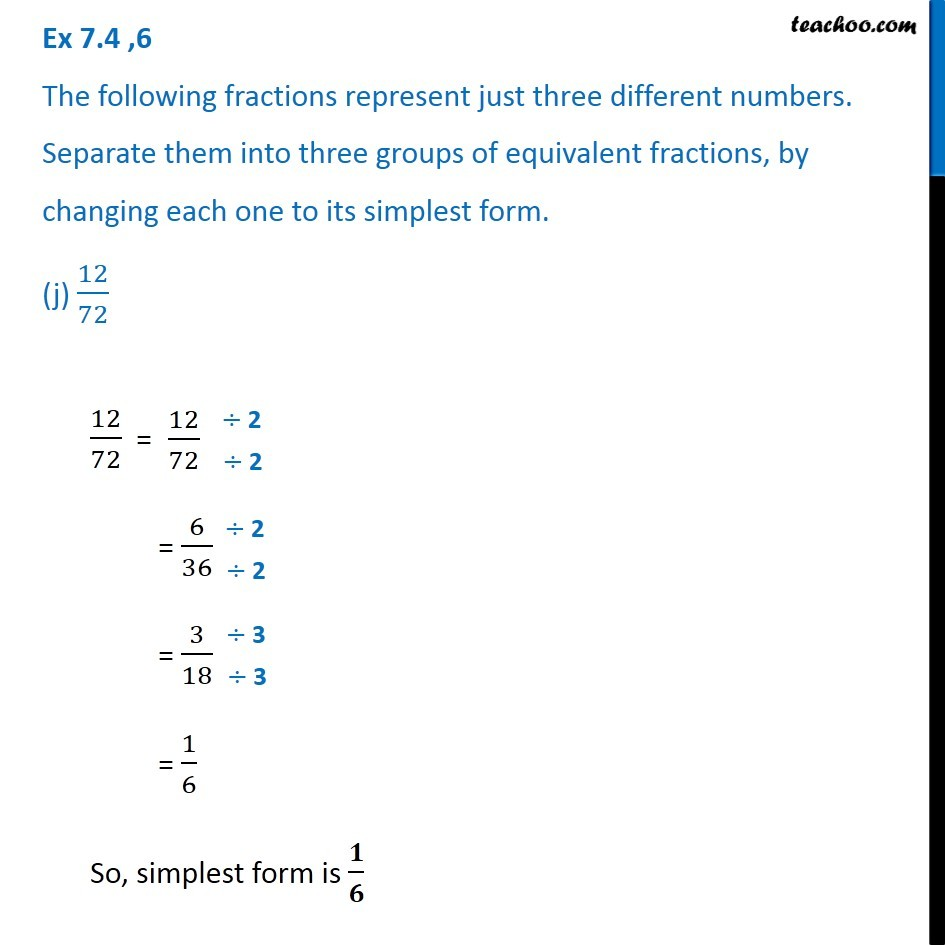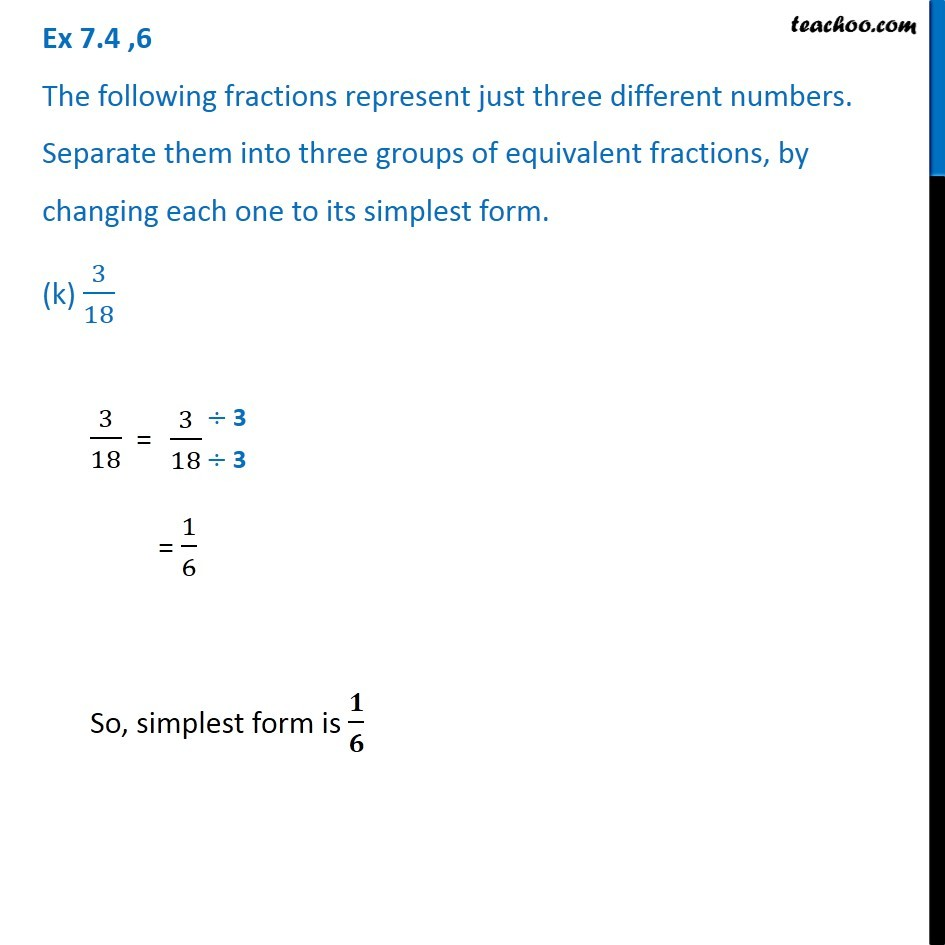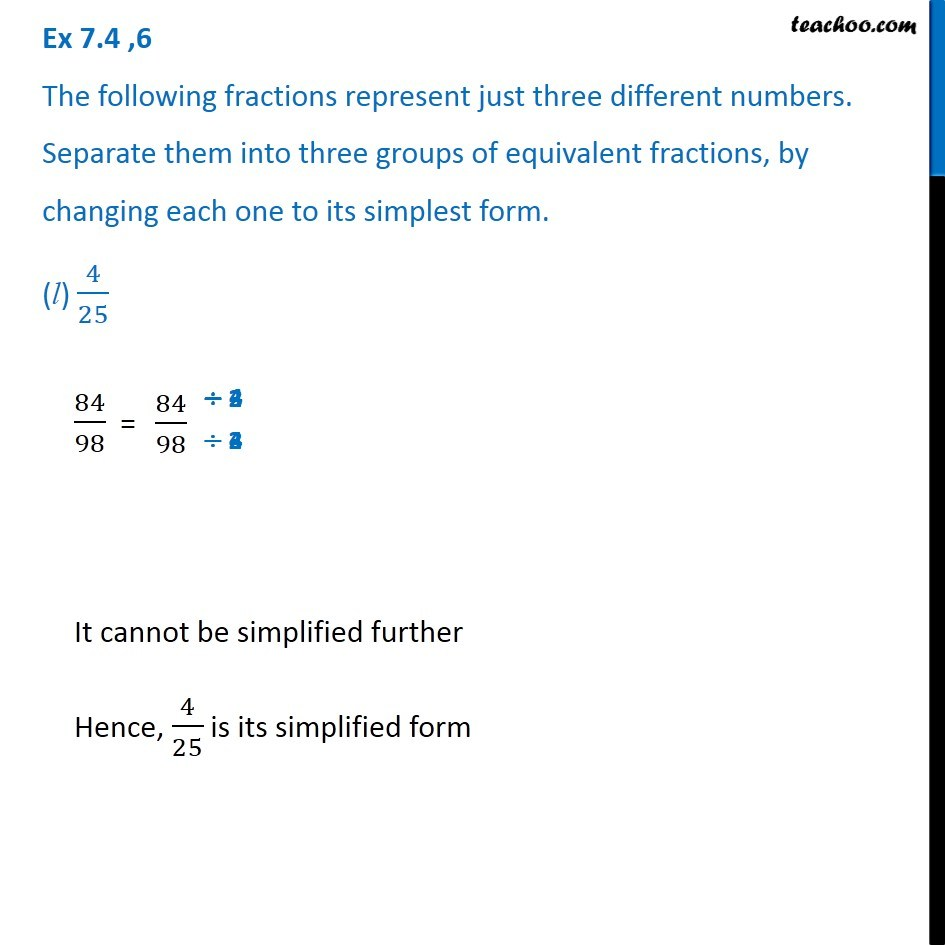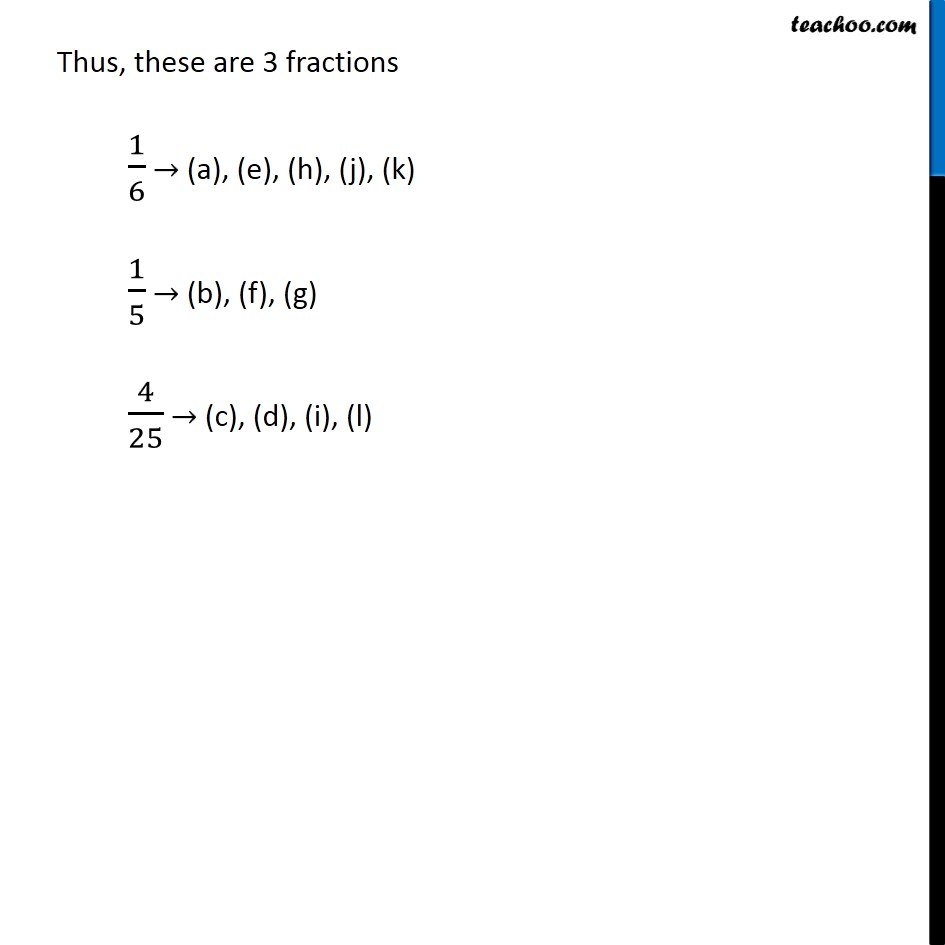Introducing your new favourite teacher - Teachoo Black, at only ₹83 per month

### Transcript

Ex 7.4 ,6 The following fractions represent just three different numbers. Separate them into three groups of equivalent fractions, by changing each one to its simplest form. (a) 2/12 We have to convert all of them into simplest from. 2/12 = = 1/6 So, simplest form is 𝟏/𝟔 Ex 7.4 ,6 The following fractions represent just three different numbers. Separate them into three groups of equivalent fractions, by changing each one to its simplest form. (b) 3/15 3/15 = = 1/5 So, simplest form is 𝟏/𝟓 Ex 7.4 ,6 The following fractions represent just three different numbers. Separate them into three groups of equivalent fractions, by changing each one to its simplest form. (c) 8/50 8/50 = = 4/25 So, simplest form is 𝟒/𝟐𝟓 Ex 7.4 ,6 The following fractions represent just three different numbers. Separate them into three groups of equivalent fractions, by changing each one to its simplest form. (d) 16/100 16/100 = = 8/50 = 4/25 So, simplest form is 𝟒/𝟐𝟓 Ex 7.4 ,6 The following fractions represent just three different numbers. Separate them into three groups of equivalent fractions, by changing each one to its simplest form. (e) 10/60 10/60 = = 1/6 So, simplest form is 𝟏/𝟔 Ex 7.4 ,6 The following fractions represent just three different numbers. Separate them into three groups of equivalent fractions, by changing each one to its simplest form. (f) 15/75 15/75 = = 5/25 = 1/5 So, simplest form is 𝟏/𝟓 Ex 7.4 ,6 The following fractions represent just three different numbers. Separate them into three groups of equivalent fractions, by changing each one to its simplest form. (g) 12/60 12/60 = = 6/30 = 3/15 = 1/5 So, simplest form is 𝟏/𝟓 Ex 7.4 ,6 The following fractions represent just three different numbers. Separate them into three groups of equivalent fractions, by changing each one to its simplest form. (h) 16/96 Ex 7.4 ,6 The following fractions represent just three different numbers. Separate them into three groups of equivalent fractions, by changing each one to its simplest form. (i) 12/75 12/75 = = 4/25 So, simplest form is 𝟒/𝟐𝟓 Ex 7.4 ,6 The following fractions represent just three different numbers. Separate them into three groups of equivalent fractions, by changing each one to its simplest form. (j) 12/72 12/72 = = 6/36 = 3/18 = 1/6 So, simplest form is 𝟏/𝟔 Ex 7.4 ,6 The following fractions represent just three different numbers. Separate them into three groups of equivalent fractions, by changing each one to its simplest form. (k) 3/18 3/18 = = 1/6 So, simplest form is 𝟏/𝟔 Ex 7.4 ,6 The following fractions represent just three different numbers. Separate them into three groups of equivalent fractions, by changing each one to its simplest form. (l) 4/25 84/98 = It cannot be simplified further Hence, 4/25 is its simplified form Thus, these are 3 fractions 1/6 → (a), (e), (h), (j), (k) 1/5 → (b), (f), (g) 4/25 → (c), (d), (i), (l)